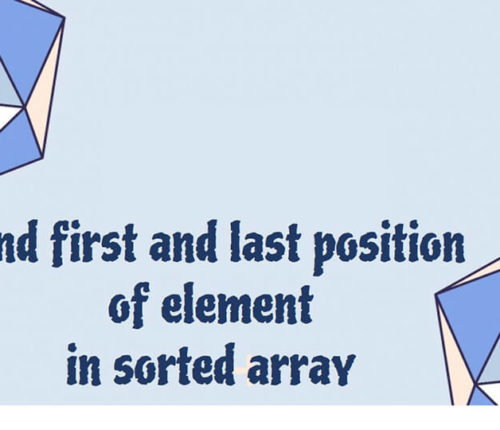# Find the Contiguous Subarray with Sum to a Given Value in an array

### Problem :

Given an array of positive integer and given value X, find Contiguous sub array whose sum is equal to X.
For example:

### Solution:

#### Solution 1:

Check all sub arrays and if current sum is equal to X, return. This will require two loops and if currentSum is greater than X tben try another sub array.
Java code:

Time Complexity : O(N^2)

#### Solution 2:

Lets say array is arr[] and given sum is X.

• Iterate over array arr[].
• If currentSum is less than X then add current element to currentSum.
• If currentSum is greater than X , it means we need to remove starting elements to make currentSum less than X.
• If CurrentSum is equal to X, we got the continuous sub array, print it.
Java Code:
Time Complexity : O(N)

### Java program to find the Contiguous Subarray with Sum to a Given Value in an array :

When you run above program , you will get below output:

import_contacts

### You may also like:## Related Posts

•04 June

### Search for a range Leetcode – Find first and last position of element in sorted array

Table of ContentsApproach 1 (Using Linear Search)Approach 2 (Using Modified Binary Search-Optimal) In this article, we will look into an interesting problem asked in Coding Interviews related to Searching Algorithms. The problem is: Given a Sorted Array, we need to find the first and last position of an element in Sorted array. This problem is […]

•28 March

### Sort an array of 0s, 1s and 2s

Table of ContentsProblemSolution If you want to practice data structure and algorithm programs, you can go through Java coding interview questions. In this post, we will see how to sort an array of 0s, 1s and 2s.We have already seen a post on sort 0s and 1s in an array. Problem Given an array containing zeroes, […]

•04 March

### Check if it is possible to reach end of given Array by Jumping

Table of ContentsProblemSolution If you want to practice data structure and algorithm programs, you can go through Java coding interview questions. ﻿ ﻿ Problem Given an array with positive integers as elements indicating the maximum length of a jump which can be made from any position in the array. Check if it is possible to have […]

•17 February

### Check if Array Elements are Consecutive

Table of ContentsProblemSolutionProgram to check if Array Elements are Consecutive If you want to practice data structure and algorithm programs, you can go through 100+ data structure and algorithm programs. In this post, we will see how to check if array elements are consecutive. Problem Given an array, we need to check if array contains […]

•01 November

### Find the local minima in array

Table of ContentsProblemSolutionNaive approachEfficient approach If you want to practice data structure and algorithm programs, you can go through Java coding interview questions. In this post, we will see how to find the local minima in the array. Problem An element is local minima if it is less than its neighbors. int [] arr = {10, […]

•22 October

### Sliding Window Maximum in java

Table of ContentsProblemSolution If you want to practice data structure and algorithm programs, you can go through Java coding interview questions. In this post, we will see about Sliding Window Maximum in java Problem Given an Array of integers and an Integer k, Find the maximum element of from all the contiguous subarrays of size K. […]

## Subscribe to our newletter

Get quality tutorials to your inbox. Subscribe now.